Courses

# Test: Fractions

## 10 Questions MCQ Test NCERT Mathematics for CAT Preparation | Test: Fractions

Description
This mock test of Test: Fractions for Class 6 helps you for every Class 6 entrance exam. This contains 10 Multiple Choice Questions for Class 6 Test: Fractions (mcq) to study with solutions a complete question bank. The solved questions answers in this Test: Fractions quiz give you a good mix of easy questions and tough questions. Class 6 students definitely take this Test: Fractions exercise for a better result in the exam. You can find other Test: Fractions extra questions, long questions & short questions for Class 6 on EduRev as well by searching above.
QUESTION: 1

### What is the fraction of the  shaded area?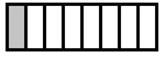Solution:

Total Blocks = 8

Hence fraction of shaded region = 1/8

QUESTION: 2

### Give a proper fraction whose numerator is 5 and denominator is 7.

Solution:

It is very obvious, as given 5 is the numerator and 7 is the denominator.

QUESTION: 3

### 2x ? - 6 = 676/26 What will come in place of question mark?

Solution:

2x ? - 6 = 676/26

2x ? - 6 = 26

2x ? = 32

? = 16

QUESTION: 4

An integer is 10 more than its one-third part. The integer is

Solution:

Let the integer be x.
x=(x/3)+10
3x=(x+30)
2x=30
x=15

QUESTION: 5

A __________ is a number representing part of a whole.

Solution:

A fraction is a number representing a part of a whole. The whole may be a single object or a group of objects.

Consider the fraction 7/12. This fraction is read as ”seven-twelfth” which means that 7 parts out of 12 equal parts in which the whole is divided. In the fraction 7/12, 7 is called the numerator and 12 is called the denominator.

QUESTION: 6

What is the fraction of the shaded area?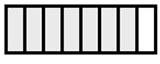Solution:

Total Blocks = 8

Hence fraction of shaded region = 7/8

QUESTION: 7

What is increasing order of the fractions 14/17, 10/12, 6/7, 18/22?

Solution: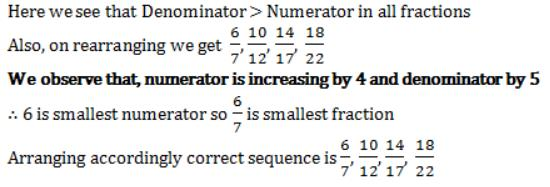QUESTION: 8

Write the fraction representing the shaded region in the below figure.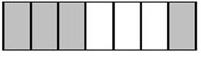Solution:

Total Blocks = 7

Hence fraction of shaded region = 4/7

QUESTION: 9

What is the fraction of the shaded area?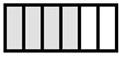Solution:

Total Blocks = 6

Hence fraction of shaded region = 4/6 = 2/3

QUESTION: 10

The simplest form of 12/20 is

Solution:
• Dividing both 12 & 20 by four we will get  3/5.